SBOS630D December   2013  – August 2016

PRODUCTION DATA.

1. Features
2. Applications
3. Description
4. Revision History
5. Pin Configuration and Functions
6. Specifications
7. Detailed Description
1. 7.1 Overview
2. 7.2 Functional Block Diagram
3. 7.3 Feature Description
4. 7.4 Device Functional Modes
8. Application and Implementation
1. 8.1 Application Information
2. 8.2 Typical Application
1. 8.2.1 TIA With Associated Signal Chain
2. 8.2.2 Extending Transimpedance Bandwidth
9. Power-Supply Recommendations
10. 10Layout
11. 11Device and Documentation Support
1. 11.1 Device Support
1. 11.1.1 Development Support
2. 11.2 Documentation Support
4. 11.4 Community Resources
6. 11.6 Electrostatic Discharge Caution
7. 11.7 Glossary
12. 12Mechanical, Packaging, and Orderable Information

• RGT|16
• RGT|16

## 6 Specifications

### 6.1 Absolute Maximum Ratings(1)

over operating free-air temperature range (unless otherwise noted)
MIN MAX UNIT
Voltage Supply voltage, VS– to VS+ 3.8 V
Input and output voltage, VIN, VOUT pins (VS–) – 0.7 (VS+) + 0.7
Differential input voltage 1
Current Output current 50 mA
Input current, VIN pin 10
Continuous power dissipation See Thermal Information table
Temperature Maximum junction, TJ 150 °C
Maximum junction, TJ (continuous operation, long-term reliability) 140
Operating free-air, TA –40 85
Storage, Tstg –65 150
(1) Stresses beyond those listed under absolute maximum ratings may cause permanent damage to the device. These are stress ratings only, and functional operation of the device at these or any other conditions beyond those indicated under recommended operating conditions is not implied. Exposure to absolute-maximum-rated conditions for extended periods may affect device reliability.

### 6.2 ESD Ratings

VALUE UNIT
V(ESD) Electrostatic discharge Human-body model (HBM), per ANSI/ESDA/JEDEC JS-001(1) ±2000 V
Charged-device model (CDM), per JEDEC specification JESD22-C101(2) ±500
(1) JEDEC document JEP155 states that 500-V HBM allows safe manufacturing with a standard ESD control process.
(2) JEDEC document JEP157 states that 250-V CDM allows safe manufacturing with a standard ESD control process.

### 6.3 Recommended Operating Conditions

over operating free-air temperature range (unless otherwise noted)
MIN NOM MAX UNIT
VSS Supply input voltage 2.7 3.3 3.6 V
TJ Operating junction temperature –40 85 °C

### 6.4 Thermal Information

THERMAL METRIC(1) OPA857 UNIT
RGT (VQFN)
16 PINS
RθJA Junction-to-ambient thermal resistance 67.1 °C/W
RθJC(top) Junction-to-case(top) thermal resistance 91.6 °C/W
RθJB Junction-to-board thermal resistance 41.6 °C/W
ψJT Junction-to-top characterization parameter 7.1 °C/W
ψJB Junction-to-board characterization parameter 41.7 °C/W
RθJC(bot) Junction-to-case(bottom) thermal resistance 23.1 °C/W

### 6.5 Electrical Characteristics

at TA = 25°C(2), VS = 3.3 V, VS+ – VS– = 3.3 V, CSource = 1.5 pF, VOUT = 0.5 VPP (differential), RL = 500-Ω differential, single-ended input, pseudo-differential output, and input and output referenced to midsupply (unless otherwise noted)
PARAMETER TEST CONDITIONS MIN TYP MAX UNIT TEST LEVEL(1)
AC PERFORMANCE
Small-signal bandwidth CTRL = 1, TA = –40°C to +85°C 105 MHz C
CTRL = 0, TA = –40°C to +85°C 125 MHz C
SR Slew rate (differential) VOUT = 1-V step 215 V/μs C
tS Settling time to 1% VOUT = 0.5-V step, CTRL = 0, TA = 25°C 8 ns B
VOUT = 0.5-V step, CTRL = 1, TA = 25°C 8 ns B
Settling time to 0.001% VOUT = 0.5-V step, CTRL = 0 600 ns C
VOUT = 0.5-V step, CTRL = 1 700 ns C
HD2 Second-harmonic distortion VOUT = 0.5 VPP, f = 10 MHz, RF = 5 kΩ, TA = 25°C –80 dBc C
VOUT = 0.5 VPP, f = 10 MHz, RF = 20 kΩ, TA = 25°C –83 dBc C
HD3 Third-harmonic distortion VOUT = 0.5 VPP, f = 10 MHz, RF = 5 kΩ, TA = 25°C –88 dBc C
VOUT = 0.5 VPP, f = 10 MHz, RF = 20 kΩ, TA = 25°C –83 dBc C
Equivalent input-referred current noise CTRL = 0, using 135-MHz brickwall filter 25 nARMS C
CTRL = 1, using 135-MHz brickwall filter 15 nARMS C
Overdrive recovery time IIN = 2x overload, CTRL = 1, settling to 1% of final value 25 ns B
Closed-loop output impedance f = 1 MHz (differential) 50 Ω C
DC PERFORMANCE
Transimpedance gain CTRL = 1 into 500 Ω(4)(5) 18.2 C
CTRL = 0 into 500 Ω(4)(5) 4.5 C
Transimpedance gain error TA = 25°C, RF = 20 kΩ and RF = 5 kΩ ±1% ±15% A
VOO Output offset voltage TA = +25°C ±1 ±5 mV A
TA = –40°C to +85°C(3) ±6 mV B
Output offset voltage drift TA = –40°C to +85°C(3) ±15 μV/°C C
VICR Common-mode voltage range TA = 25°C, OUTN 1.78 1.83 1.88 V A
INPUT
Input pin capacitance 2 pF C
OUTPUT
Output voltage swing OUT, TA = 25°C 0.6 1.9 V A
TA = –40°C to +85°C(3) 1.9 V B
Output current drive
(for linear operation)
OUT, differential 50-Ω between OUT and OUTN +5 mA C
–20 mA C
POWER SUPPLY
Quiescent operating current CTRL = 0, TA = 25°C 20.5 23.4 26.3 mA A
CTRL = 0, TA = –40°C to +85°C(3) 20.0 26.8 mA B
CTRL = 1, TA = 25°C 20.5 23.4 26.3 mA A
CTRL = 1, TA = –40°C to +85°C(3) 20.0 26.8 mA B
PSRR Power-supply rejection ratio At dc, TA = 25°C 70 80 dB A
f = 10 MHz, TA = –40°C to +85°C(3) 15 18 dB B
LOGIC LEVEL (CTRL)
VIH High-level input voltage 2 V A
VIL Low-level input voltage 0.8 V A
High-level control pin input bias current 1 µA A
Low-level control pin input bias current 1 µA A
(1) Test levels: (A) 100% tested at 25°C. Overtemperature limits set by characterization and simulation. (B) Limits set by characterization and simulation. (C) Typical value only for information.
(2) Junction temperature = ambient for 70°C specifications.
(3) Junction temperature = ambient at low temperature; junction temperature = ambient + 3.5°C for overtemperature specifications.
(4) See the Application and Implementation section for details on loading and effective transimpedance gain.
(5) Note that the effective transimpedance gain is reduced to 18.2 kΩ and 4.5 kΩ, respectively, with a 500-Ω load resulting from the internal series resistance on OUT and OUTN.

### 6.6 Typical Characteristics

At TA = 25°C, CS = 1.5 pF, and RL = 500-Ω differential between OUT and OUTN (unless otherwise noted).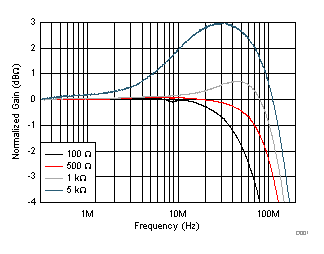TZ Gain = 20 kΩ
Figure 1. Frequency Response vs Load Resistance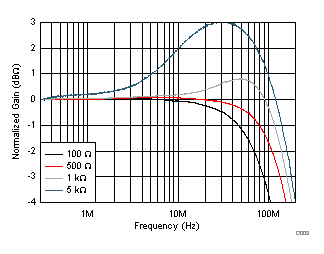TZ Gain = 5 kΩ
Figure 3. Frequency Response vs Load Resistance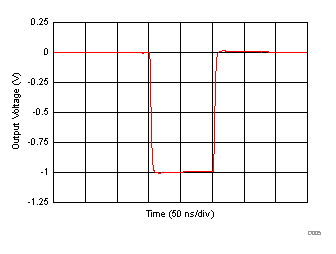TZ Gain = 20 kΩ
Figure 5. 1-VPP Pulse Response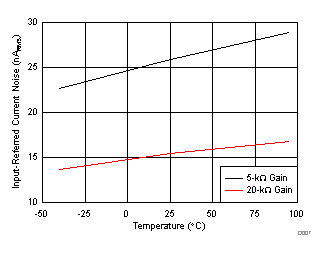Figure 7. RMS Input-Referred Current Noise
vs Temperature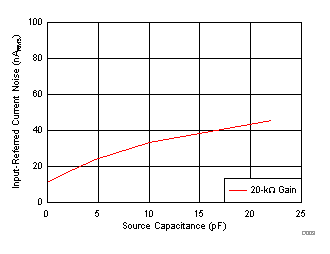Figure 9. RMS Input-Referred
Current Noise vs Capacitance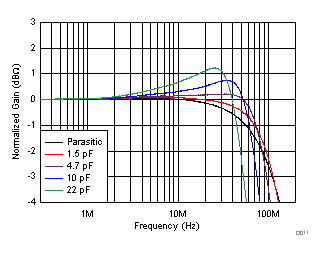TZ Gain = 20 kΩ
Figure 11. Gain Frequency Response vs
Input Capacitance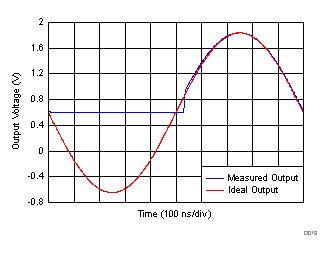TZ Gain = 20 kΩ
Figure 13. 2x Overdrive Recovery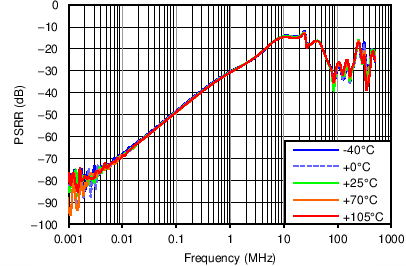TZ Gain = 20-kΩ
Figure 15. Power-Supply Rejection Ratio
vs Frequency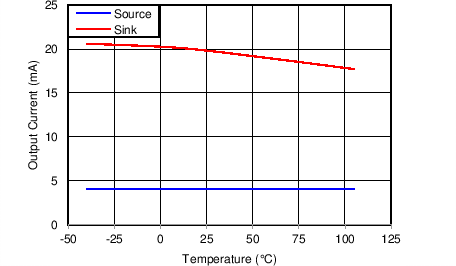Figure 17. Output Current vs Temperature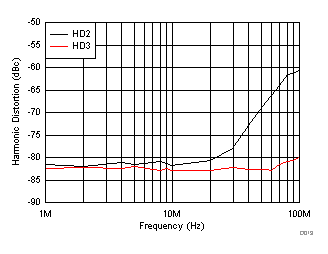TZ Gain = 20 kΩ, RLOAD = 500 Ω
Figure 19. Harmonic Distortion vs FrequencyTZ Gain = 20 kΩ, RLOAD = 500 Ω, f = 50 MHz
Figure 21. Harmonic Distortion vs Output Voltage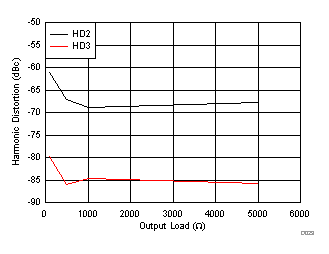TZ Gain = 20 kΩ, f = 50 MHz
Figure 23. Harmonic Distortion vs RLOADTZ Gain = 20 kΩ, TA = 25°C, RLOAD = 500 Ω, f = 50 MHz
Figure 25. Harmonic Distortion vs Supply Voltage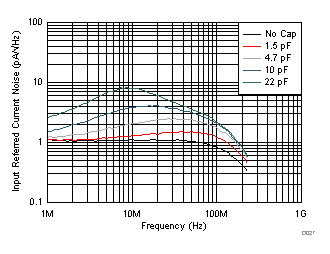TZ Gain = 20 kΩ
Figure 27. Input-Referred Current Noise Density vs Frequency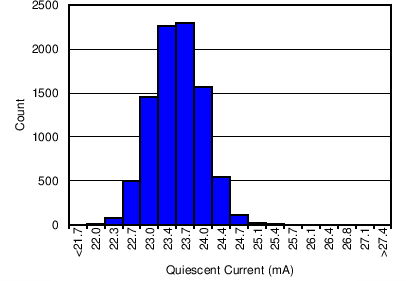TZ Gain = 5 kΩ
Figure 29. IQ Histogram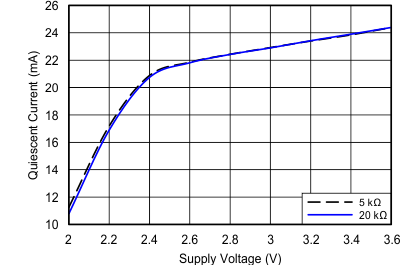Figure 31. Quiescent Current vs Supply Voltage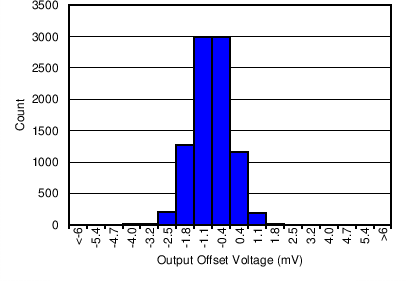TZ Gain = 20 kΩ
Figure 33. Differential VOSO HistogramFigure 35. Reference Voltage (VOUTN) Distribution Histogram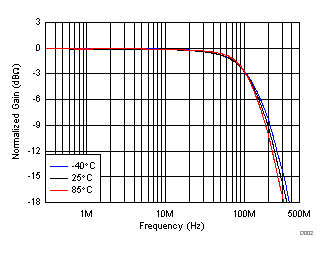TZ Gain = 20 kΩ
Figure 2. Frequency Response vs Temperature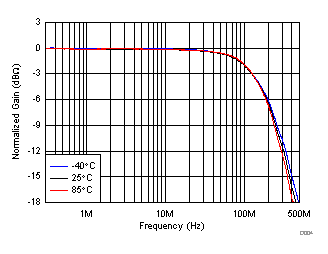TZ Gain = 5 kΩ
Figure 4. Frequency Response vs Temperature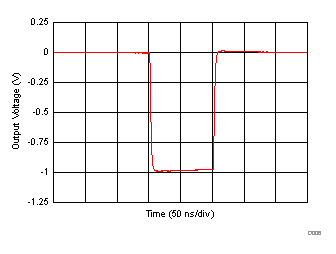TZ Gain = 5 kΩ
Figure 6. 1-VPP Pulse Response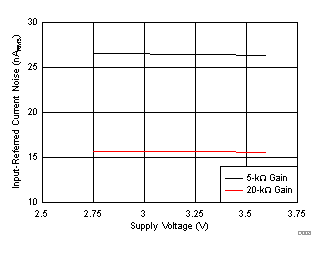Figure 8. RMS Input-Referred Current Noise
vs Supply Voltage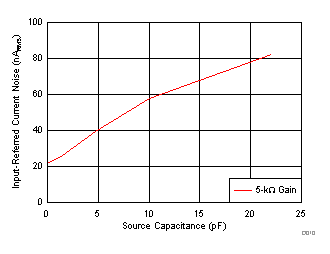Figure 10. Gain RMS Input-Referred
Current Noise vs Input Capacitance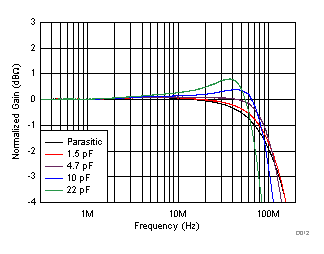TZ Gain = 5 kΩ
Figure 12. Gain Frequency Response vs
Input Capacitance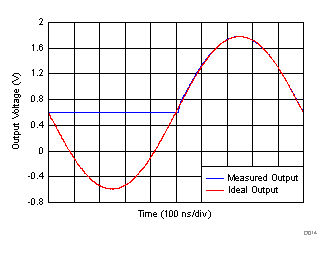TZ Gain = 5 kΩ
Figure 14. 2x Overdrive Recovery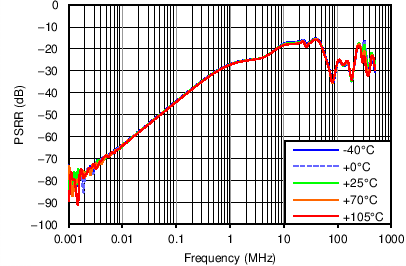TZ Gain = 5 kΩ
Figure 16. Power-Supply Rejection Ratio
vs Frequency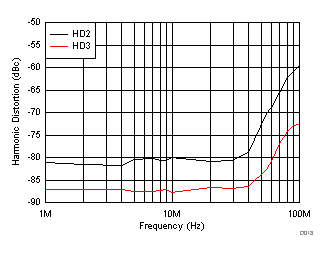TZ Gain = 5 kΩ, RLOAD = 500 Ω
Figure 18. Harmonic Distortion vs Frequency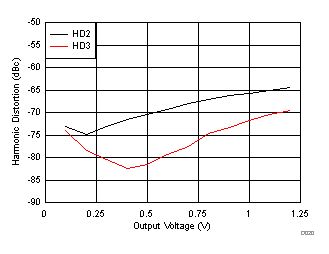TZ Gain = 5 kΩ, RLOAD = 500 Ω, f = 50 MHz
Figure 20. Harmonic Distortion vs Output Voltage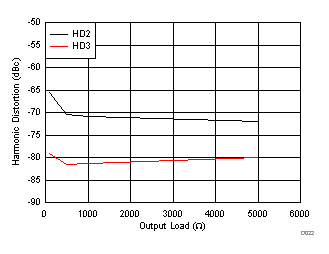TZ Gain = 5 kΩ, f = 50 MHz
Figure 22. Harmonic Distortion vs RLOAD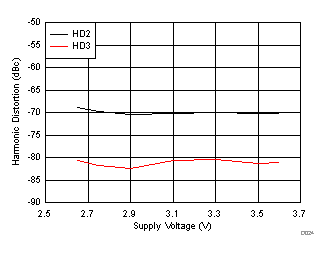TZ Gain = 5 kΩ, TA = 25°C, RLOAD = 500 Ω, f = 50 MHz
Figure 24. Harmonic Distortion vs Supply VoltageTZ Gain = 5 kΩ
Figure 26. Input-Referred Current Noise Density vs Frequency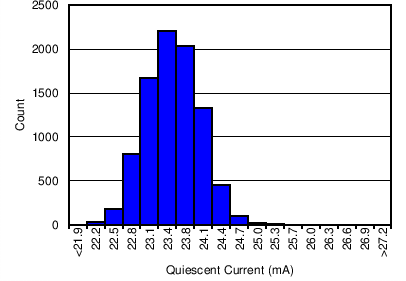TZ Gain = 20 kΩ
Figure 28. IQ Histogram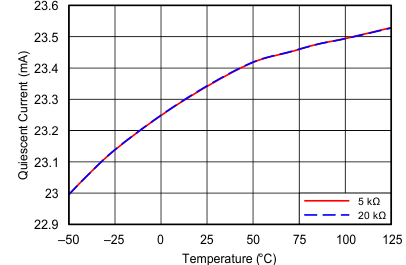Figure 30. Quiescent Current vs Temperature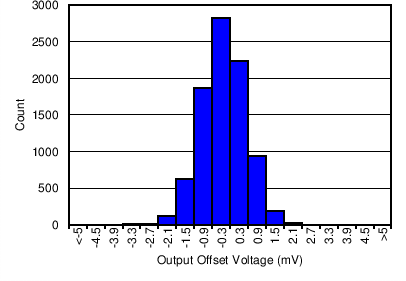TZ Gain = 5 kΩ
Figure 32. Differential VOSO Histogram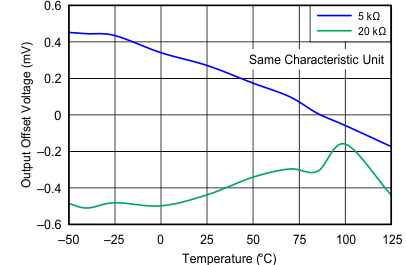Figure 34. Output Offset Voltage vs Temperature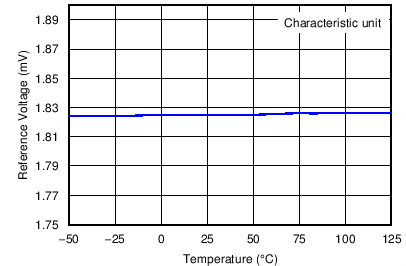Figure 36. Reference Voltage (VOUTN) vs Temperature